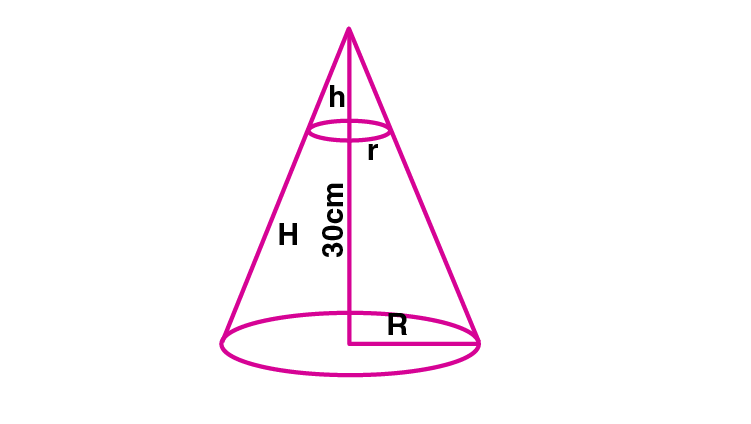Newbie

# The height of a cone is 30 cm. A small cone is cut off at the top by a plane parallel to its base. If its volume be 1/27 of the volume of the given cone, at what height above the base is the section cut?

• 0

This question is Based on Mensuration Chapter of M.L Aggarwal book for ICSE BOARD for class 10.
Here the height of a cone is given. A small cone is cut off at the top by a plane parallel to its base. If its volume be 1/27 of the volume of the given cone, at what height above the base is the section cut?
This is the Question Number 18, Exercise 17.2 of M.L Aggarwal.

Share

1.Given height of the cone, H = 30 cm

Let R be the radius of the given cone and r be radius of small cone.

Let h be the height of small cone.Volume of the given cone = (1/3)R2H

Volume of the small cone = 1/27 th of the volume of the given cone.

(1/3)r2h = (1/27)× (1/3)R2H

Substitute H = 30

(1/3)r2h = (1/27)× (1/3)R2×30

r2h/R2 = 30/27

r2h/R2 = 10/9 ….(i)

From figure, r/R = h/H

r/R = h/30 ….(ii)

Substitute (ii) in (i)

(h/30)2×h = 10/9

h3/900 = 10/9

h3 = 900×10/9 = 1000

Taking cube root on both sides.

h = 10 cm

H-h = 30-10 = 20

The small cone is cut at a height of 20 cm above the base.

• 0